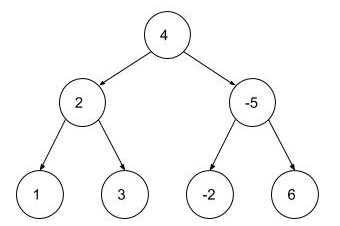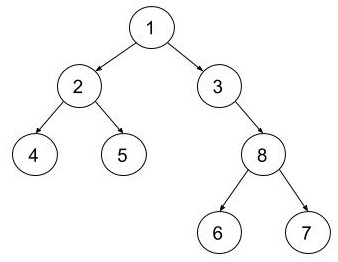New update is available. Click here to update.Close
Topic list
Maximum Level Sum
EASY
15 mins2 upvotes
Trees
Topics (Covered in this problem)
Problem solved
Skill meterTrees
-
-
Other topics
Problem solved
Skill meterStrings
-
-Matrices (2D Arrays)
-
--
-Sorting
-
-Binary Search
-
-Stacks & Queues
-
-Graph
-
-Dynamic Programming
-
-Greedy
-
-Tries
-
-Arrays
-
-SQL
-
-Binary Search Trees
-
-Heap
-
-Bit Manipulation
-
-
Solve problems & track your progress
Checkout your overall progress in every topic here
BecomeSensei
in DSA topics
Open the topic and solve more problems associated with it to improve your skills
Check out the skill meter for every topic
See how many problems you are left with to solve for cracking any stage. Score more than zero to get your progress counted.

# Maximum Level Sum

Easy0/40
Avg time to solve 15 mins
Success Rate 80 %Share2 upvotes

## Problem Statement

#### Given a Binary Tree with integer nodes, your task is to find the maximum level sum among all the levels in the Binary Tree. The sum of any level in a tree is the sum of all the nodes present at that level.

Detailed explanation ( Input/output format, Notes, Constraints, Images )##### Sample Input 1:
``````1
4 2 -5 1 3 -2 6 -1 -1 -1 -1 -1 -1 -1 -1
``````
##### Sample Output 1:
``````8
``````
##### Explanation of Sample Output 1:``````The Sum of all nodes of the 0th level is 4.

The Sum of all nodes of  the1st level is  2 + (-5) = -3.

The Sum of all nodes of the 2nd level is 1 + 3 +(-2) + 6 = 8.

Hence, the maximum level sum is 8.
``````
##### Sample Input 2:
``````1
1 2 3 4 5 -1 8 -1 -1 -1 -1 6 7 -1 -1 -1 -1
``````
##### Sample Output 2:
``````17
``````
##### Explanation of Sample Output 2:``````Maximum sum is at level 2 i.e. 4 + 5 + 8 = 17.
``````AutoConsole